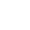﻿

/    2019-02-28

`package mainimport (    "fmt")type T struct {    string}func (t T) testT() {    fmt.Println("接受者为 T ")}func (t *T) testP() {    fmt.Println("接受者为 *T ")}func main() {    t := T{"oldboy"}    methodValue1 := t.testT    methodValue1()    methodValue2 := (&t).testT    methodValue2()    methodValue3 := t.testP    methodValue3()    methodValue4 := (&t).testP    methodValue4()}`

`package mainimport (    "fmt")type ST struct {    T}type SP struct {    *T}type T struct {    string}func (t T) testT() {    fmt.Println("类型 S 包含匿名字段 *T 或 T ，则 S 和 *S 方法集包含 T 方法")}func (t *T) testP() {    fmt.Println("类型 S 包含匿名字段 *T 或 T ，则 S 和 *S 方法集包含 *T 方法")}func main() {    st1 := ST{T{"oldboy"}}    methodValue1 := st1.testT    methodValue1()    methodValue2 := (&st1).testT    methodValue2()    methodValue3 := st1.testP    methodValue3()    methodValue4 := (&st1).testP    methodValue4()    sp1 := SP{&T{"oldboy"}}    methodValue5 := sp1.testT    methodValue5()    methodValue6 := (&sp1).testT    methodValue6()    methodValue7 := sp1.testP    methodValue7()    methodValue8 := (&sp1).testP    methodValue8()}`(0)

﻿